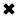# Voltage Explained#### Voltage pushes current through a wire similar to water pressure pushing water through a pipe.

Voltage (V, EMF, or E) is electrical pressure, a potential force or difference in electrical charge between two points in an electric circuit. Voltage pushes current through a wire similar to water pressure pushing water through a pipe. The voltage level or value is proportional to the difference in the electrical potential energy between two points. Voltage is measured in volts (V). A voltage of one volt is required to force one ampere of current through one ohm of resistance. The letter E, which stands for EMF (Electromotive Force), or the letter V, which stands for volt, is commonly used to represent voltage in an algebraic formula.

Voltage, or a difference in potential, exists between any two charges that are not exactly equal to each other. Even an uncharged body has a potential difference with respect to a charged body; it is positive with respect to a negative charge and negative with respect to a positive charge. Voltage also exists between two unequal positive charges or between two unequal negative charges. Therefore, voltage is purely relative and is not used to express the actual amount of charge, but rather to compare one charge to another and indicate the electromotive force between the two charges being compared.

A voltmeter is used to measure the voltage, or potential energy difference of a load or source. Voltage exists between two points and does not flow through a circuit as current does. As a result, a voltmeter is connected across, or in parallel, with the two points. Very small amounts of voltage are measured in millivolts and microvolts, whereas high-voltage values are expressed in kilovolts and megavolts.

Electrical Resistance (R) is the opposition to the flow of electrons (current). It is like electric friction that slows the flow of current. Resistance is measured in ohms. The Greek symbol Q (omega) is often used to represent ohms.

Resistance is due, in part, to each atom resisting the removal of an electron by its attraction to the positive nucleus. Collisions of countless electrons and atoms as the electrons move through the conductor create additional resistance also. The resistance created causes heat in the conductor when current flows through it; that is why a wire becomes warm when current flows through it. The higher the resistance, the greater the opposition to current flow. The elements of an electric range become hot, and the filament of an incandescent lamp becomes extremely hot because they have a much higher resistance than the conductors that carry the current to them.

Every electrical component has resistance and this resistance changes electric energy into another form of energy—heat, light, or motion.

A special meter called an ohmmeter can measure the resistance of a device in ohms when no current is flowing. A standard multimeter contains an ohmmeter that operates by a battery located inside the instrument. For this reason ohmmeters should never be connected to live circuits! The ease with which electric current flows through a material depends on whether there are relatively large numbers of free electrons. A material with few electrons would have a substantial resistance or opposition to current flow.

##### Basic Electricity Handbook, Vol. 1• FULLY ILLUSTRATED
• GREAT FOR SCHOOL PROJECTS
• GREAT PRICE: \$5.99
• ...

This 100+ page e-book is a great guide for those who have a basic interest in the field of electricity. This well-illustrated e-book, coupled with some basic knowledge of electricity, will give you a broad theoretical background in this fundamental subject.

CONTENTS
• FUNDAMENTALS OF ELECTRICITY
• HOW DOES ELECTRICITY WORK?
• HOW IS ELECTRICITY GENERATED?
• WHERE DOES ELECTRICITY COME FROM?
• BASIC HOME WIRING
• ELECTRICITY FOR STUDENTS
• ENERGY SAVING TIPS

##### TRAINING EF COURSES

Content Community Connection
Top